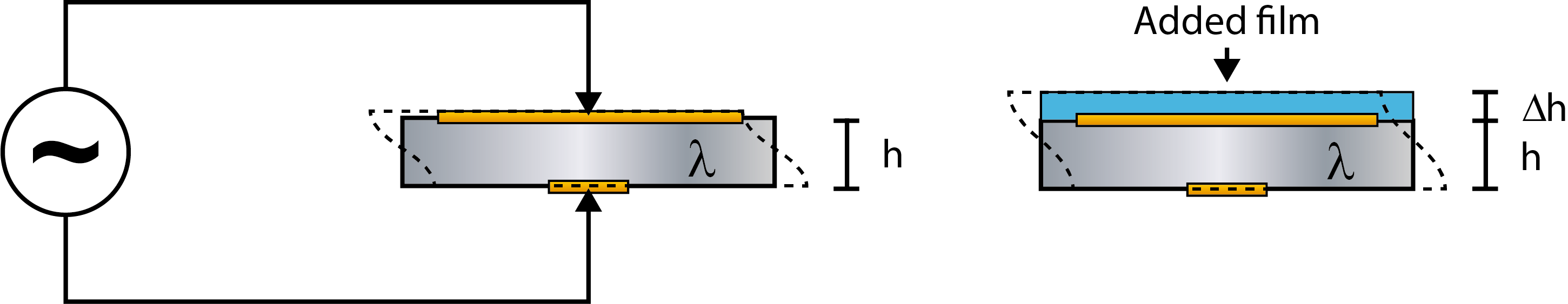{% import 'custom/page/settings/int-website.html' as config %}
{%- if content.absolute_url|replace('https:', '')|replace('http:', '') == config.homeUrl -%} {%- set homeClass = homeClass + "active-branch active" -%} {%- elif content.absolute_url is string_containg config.homeUrl -%} {%- set homeClass = "active-branch" -%} {%- endif -%}{%- if content.absolute_url == config.menuItemLogIn.url -%} {%- set loginItemClass = " active-branch active" -%} {%- elif (contentSlug) and (config.menuItemLogIn.url is string_containing contentSlug) -%} {%- set loginItemClass = " active-branch" -%} {%- endif -%}{%- if content.absolute_url == config.menuItemContact.url -%} {%- set contactItemClass = " active-branch active" -%} {%- elif (contentSlug) and (config.menuItemContact.url is string_containing contentSlug) -%} {%- set contactItemClass = " active-branch" -%} {%- endif -%}
Malin Edvardsson Jul 3, ’18 {{ blog_macros.outputReadDuration(content) }}

# What is the Sauerbrey equation?

## Surface Science Blog

In the context of QCM technology, there is often a reference to the so-called Sauerbrey equation. But what is this equation, and when should it be used?

## The Sauerbrey equation is used to calculate the mass of thin layers

The ability of the QCM technology to measure mass changes originates in the discovery of the relation between the resonance frequency and the mass. The relation was formulated in an equation in 1959 by Günter Sauerbrey, at the time a Ph.D. student in Berlin. The equation is a linear relationship between the resonance frequency of an oscillating quartz crystal and mass changes, Eq. 1.

Δm = − C ·Δf/n           (1)

In practice, this equation can be used to calculate the mass of thin layers added to a QCM crystal surface. By inserting the resonance frequency change, ∆f, that results from the layer addition, into Eq. 1, you will get the mass, ∆m, of the added layer. The constant, C, is related to the properties of quartz. For a 5 MHz crystal, C equals 17.7 ng/(cm-2∙ Hz). The parameter n is the odd overtone number, and can be 1, 3 ,5 ,7 ,… etc.

## When should the Sauerbrey equation be used?

The linear frequency-mass relation is based on the behavior of a pure quartz crystal, where a crystal of thickness h (and mass m) will have a certain resonance frequency (Fig. 1, left). The scenario where a layer of material, different from quartz, is added to the surface, can be approximated with a thicker crystal and must therefore be similar enough to the “pure-quartz” scenario. The model assumes that the thin, added layer can be approximated to be a part of the oscillating crystal itself, now with thickness h + Δh (Fig. 1, right). Therefore, the layer on the sensor must be thin, rigid and firmly attached to the crystal surface, and the equation should only be used when these conditions are fulfilled. If the layer on the sensor is soft, thick or not coupled to the surface, the equation is not valid. In these situations, the model will fail, and the calculated mass will be underestimated.Figure 1. (Left) Schematic illustration of a quartz crystal, of thickness h and mass m, excited to resonance by the application of an alternating voltage.
(Right) An oscillating crystal with added mass. Now the thickness is h + Δh and the mass is m + Δm.

## How do I know if I can use the Sauerbrey equation or not?

If you are using a QCM setup that measures the energy loss, e.g. QCM-D, or has the capability of measuring at multiple harmonics, the applicability of the Sauerbrey equation can be identified by looking at the raw data. The raw data will reveal applicability either by showing a low energy loss or overlapping overtones if those are measured. If the Sauerbrey equation is not applicable, then you need to use a model that better describes your layer, perhaps a viscoelastic model, for layer characterization.{%- set subscribeTitle = config.label.subscribeTitle -%}{%- set subscribeDesc = config.label.subscribeDesc -%}{%- set subscribeThankYou = config.label.subscribeThankYou -%}

## {{ subscribeTitle }}

{%- if subscribeDesc -%}

{{ subscribeDesc }}

{%- endif -%}
{{ "{%- blog_subscribe \"blog_subscribe\" select_blog='default', title='', response_message='" + subscribeThankYou|replace("'", '\\\'') + "', label='Blog Email Subscription', overrideable=False -%}" }}